Test: Recurrence & Searching- 1

# Test: Recurrence & Searching- 1

Test Description

## 10 Questions MCQ Test GATE Computer Science Engineering(CSE) 2023 Mock Test Series | Test: Recurrence & Searching- 1

Test: Recurrence & Searching- 1 for Computer Science Engineering (CSE) 2023 is part of GATE Computer Science Engineering(CSE) 2023 Mock Test Series preparation. The Test: Recurrence & Searching- 1 questions and answers have been prepared according to the Computer Science Engineering (CSE) exam syllabus.The Test: Recurrence & Searching- 1 MCQs are made for Computer Science Engineering (CSE) 2023 Exam. Find important definitions, questions, notes, meanings, examples, exercises, MCQs and online tests for Test: Recurrence & Searching- 1 below.
Solutions of Test: Recurrence & Searching- 1 questions in English are available as part of our GATE Computer Science Engineering(CSE) 2023 Mock Test Series for Computer Science Engineering (CSE) & Test: Recurrence & Searching- 1 solutions in Hindi for GATE Computer Science Engineering(CSE) 2023 Mock Test Series course. Download more important topics, notes, lectures and mock test series for Computer Science Engineering (CSE) Exam by signing up for free. Attempt Test: Recurrence & Searching- 1 | 10 questions in 30 minutes | Mock test for Computer Science Engineering (CSE) preparation | Free important questions MCQ to study GATE Computer Science Engineering(CSE) 2023 Mock Test Series for Computer Science Engineering (CSE) Exam | Download free PDF with solutions
 1 Crore+ students have signed up on EduRev. Have you?
*Answer can only contain numeric values
Test: Recurrence & Searching- 1 - Question 1

### The given diagram shows the flowchart for a recursive function  A(n). Assume that all statements, except for the recursive calls, have O(1) time complexity. If the worst case time complexity of this function is O(nα) then the least possible value (accurate up to two decimal positions) of α is ________. Flow chart for Recursive Function A(n).Detailed Solution for Test: Recurrence & Searching- 1 - Question 1

If they are asking for worst case complexity hence,

By calling A(n) we get A(n/2) 5 times,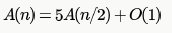Hence by applying masters theorem,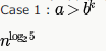Thus value of alpha will be 2.32

Test: Recurrence & Searching- 1 - Question 2

### The recurrence relation that arises in relation with the complexity of binary search is:

Detailed Solution for Test: Recurrence & Searching- 1 - Question 2

It is B. searching for only one half of the list. leading to T(n/2) + constant time in comparing and finding mid element.

Test: Recurrence & Searching- 1 - Question 3

### The recurrence relation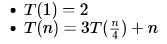has the solution T (n) equal to

Detailed Solution for Test: Recurrence & Searching- 1 - Question 3

According to Master theorem,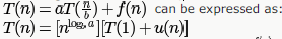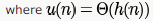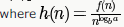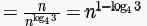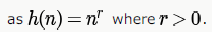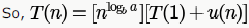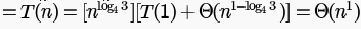Test: Recurrence & Searching- 1 - Question 4

Let

T(n) be the function defined by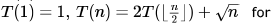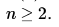Which of the following statements is true?

Detailed Solution for Test: Recurrence & Searching- 1 - Question 4

using master method (case 1)Test: Recurrence & Searching- 1 - Question 5

The solution to the recurrence equation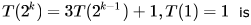Detailed Solution for Test: Recurrence & Searching- 1 - Question 5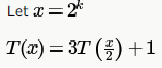We can apply Master's theorem case 1 with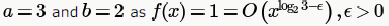So,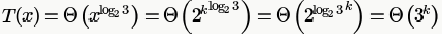So, only option possible is B.

We can also directly solve as follows: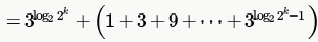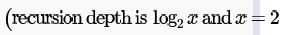OR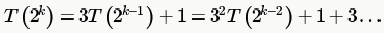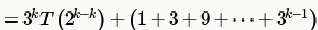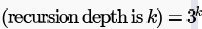Test: Recurrence & Searching- 1 - Question 6

The running time of the following algorithm

Procedure A(n)

If n<=2 return (1) else return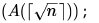is best described by:

Detailed Solution for Test: Recurrence & Searching- 1 - Question 6

The complexity will be the number of times the recursion happens which is equal to the number of times we can take square root of n recursively, till n becomes 2.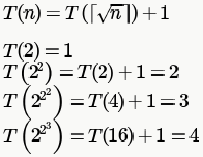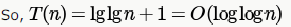Test: Recurrence & Searching- 1 - Question 7

Consider the following recurrence relation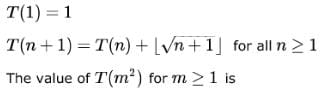Detailed Solution for Test: Recurrence & Searching- 1 - Question 7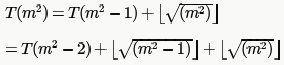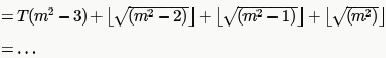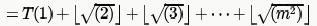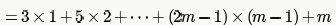(We are taking floor of square root of numbers, and between
successive square roots number of numbers are in the series 3,5,7... like 3 numbers from 1...4,5numbers from 5-9 and so on).

We can try out options here or solve as shown at end:

Put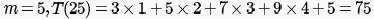Test: Recurrence & Searching- 1 - Question 8

The recurrence equation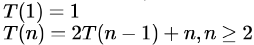evaluates to

Detailed Solution for Test: Recurrence & Searching- 1 - Question 8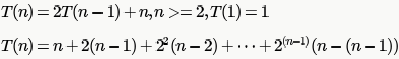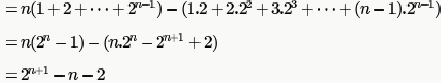Test: Recurrence & Searching- 1 - Question 9

Consider a list of recursive algorithms and a list of recurrence relations as shown below. Each recurrence relation corresponds to exactly one algorithm and is used to derive the time complexity of the algorithm.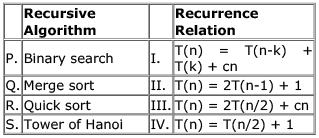Which of the following is the correct match between the algorithms and their recurrence relations?

Detailed Solution for Test: Recurrence & Searching- 1 - Question 9

These are examples of some standard algorithms whose
Merge Sort:    T(n) = 2T(n/2) + Θ(n). It falls in case 2 as c is 1 and Logba] is also 1 and  the solution is Θ(n Logn) //time complexity can be evaluated using Master Method

Binary Search: T(n) = T(n/2) + Θ(1). It also falls in case 2 as c is 0 and Logba is also 0 and the solution is Θ(Logn) //time complexity can be evaluated using Master Method

Quick Sort : Time taken by QuickSort in general can be written as  T(n) = T(k) + T(n-k-1) +(n)

Tower of Hanoi : T(n) = 2T(n-1) + 1

Test: Recurrence & Searching- 1 - Question 10

Let T(N) be a function defined by the recurrence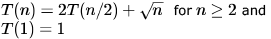Which of the following statements is TRUE?

Detailed Solution for Test: Recurrence & Searching- 1 - Question 10

Option C it can be done by Master theorem.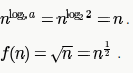So,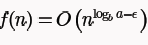is true for any real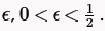Hence Master theorem Case 1 satisfied,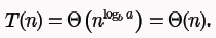## GATE Computer Science Engineering(CSE) 2023 Mock Test Series

150 docs|215 tests
Information about Test: Recurrence & Searching- 1 Page
In this test you can find the Exam questions for Test: Recurrence & Searching- 1 solved & explained in the simplest way possible. Besides giving Questions and answers for Test: Recurrence & Searching- 1, EduRev gives you an ample number of Online tests for practice

## GATE Computer Science Engineering(CSE) 2023 Mock Test Series

150 docs|215 tests(Scan QR code)